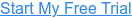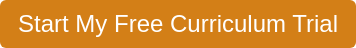The Coding Fundamentals module will help students understand the fundamental concepts of computer science and coding. Students will learn vocabulary and concepts within the lessons. Many of the lessons include mini-projects where students will complete coding tasks using a drag/drop block coding language.

### Explore Activity: Creating an Algorithm

In this exercise, students will create algorithms for two defined activities.

### Unit1:Introduction to Coding

The Introduction to Coding unit gives students a peek into coding as a profession. Coding is clearly defined in this unit. In addition, students are introduced to some common careers in the field, as well as given a behind-the-scenes look at how computers use code to function.

#### Lessons

1. What is Coding?
2. Careers in Coding
3. The Secret Life of Code

### Unit2:Basics of Coding

In Basics of Coding, we break down the programming process to prepare students to tackle real-world problems using coding. Students also begin to learn various elements of coding like commands, command statements, loops, and conditional statements.

#### Lessons

1. Hello, World
2. The Programming Process
3. Here We Go Loopty Loop
4. On One Condition

### Unit3:Diving into Coding

Diving into Coding exposes students to more difficult elements of coding, such as, events, variables, and operators.

#### Lessons

1. In Any Event
2. Variability of Variables
3. Calling all Operators

### Reflection Activity: Uses of Coding

In this exercise, students will reflect on what they have learned about coding.

### Reinforcement Activity: Coding Skills

In this exercise, students practice using various elements of coding.

### Reinforcement Activity: Current Event

Students will review articles for information related to a topic in the module. Once an article is found, students complete a Current Event form.